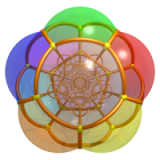# Coxeter 群基础知识 （一）：反射

1. 两个反射生成的 Coxeter 群。
2. 一般 Coxeter 群的几何实现。
3. 双曲 Coxeter 群与圆堆。
4. Coxeter 群的极小根与正则语言。

1. $V$ 上群在 Tits 锥上是如何作用的。
2. $V^\ast$ 上根系 $\Phi\in V^\ast$ 的结构。

# 单个反射

$\alpha\in V^\ast$ 是一个线性泛函，对 $v\in V$，我们用双线性对 $\langle \,,\,\rangle$ 来表示 $\alpha(v)=\langle \alpha,\,v\rangle$。这种记号有个明显的好处是它关于两个分量都是线性的，而且由于 $V$$V^\ast$ 互为对偶空间所以还是对称的，即 $\alpha$$v$ 在括号中的顺序并不重要。

$\alpha^\vee\in V$ 使得 $\langle \alpha,\,\alpha^\vee\rangle=2$$V$ 上的线性变换 $s(v) = v - \langle \alpha,\,v\rangle\alpha^\vee,\quad v\in V.$ 满足如下性质：

1. $s$ 保持 $n-1$ 维的超平面 $\alpha=0$ 不变；
2. $s$ 将非零向量 $\alpha^\vee$ 映射为 $-\alpha^\vee$
3. $s^2=1$。（这一点可以由 1, 2 推出）# 两个反射

$s_{\alpha,\alpha^\vee},\,t_{\beta,\beta^\vee}$ 是两个反射，且线性泛函 $\alpha,\beta$ 是线性无关的。考虑 $s,t$$\mathrm{GL}(V)$ 中生成的子群 $W_{s,t}$，记 $K=\{\alpha>0\}\cap\{\beta>0\}$$\alpha,\beta$ 的正半空间的交。我们希望 $W_{s,t}$$V$ 上的作用有如下性质：

1. $K\ne\emptyset$。（由于我们假定了 $\alpha,\beta$ 是线性无关的泛函，所以这一点其实是自动满足的）
2. 对任何 $w\in W_{s,t},\,w\ne1$$wK\cap K=\emptyset$

“离散地作用”翻译成万花筒的语言，就是原像 $K$ 与其所有虚像都不发生重叠。

$H_\alpha=\{\alpha=0\},\,H_\beta=\{\beta=0\}$$H_\alpha,\,H_\beta$ 都是 $n-1$ 维超平面，由于 $\alpha,\beta$ 线性无关所以 $H=H_\alpha\cap H_\beta$$n-2$ 维超平面，$s,t$ 均保持 $H$ 不动。在商空间 $V/H$ 中，$\alpha,\beta$ 仍然是两个线性无关的泛函，$H_\alpha,H_\beta$ 是两条不同的直线，$K/H$ 是这两条直线所夹的一个锥形区域。

$\Rightarrow$：若不然，$K/H$ 不是基本区域，则存在 $w\ne 1$ 使得 $w(K/H)\cap K/H$ 非空，即存在 $x,y\in K$ 使得 $w (x + H) = y + H$, 从而 $wx - y \in H$。于是 $\alpha(wx)=\alpha(y) > 0$$\beta(wx) = \beta(y) > 0$，即 $wx\in K$，这与 $K$$V$ 中的基本区域矛盾。

$\Leftarrow$：若不然，$K$ 不是基本区域，则存在 $w\ne1,x\in K$ 使得 $wx\in K$，从而 $wx + H \in K/H$，这与 $K/H$$V/H$ 中的基本区域矛盾。

1. $c_{s,t}$$c_{t,s}$ 同时为 0 或者同时小于 0。
2. 要么 $W_{s,t}$ 是有限群且 $n_{s,t}=4\cos^2(\pi/m)$，其中 $m$$\geq2$ 的正整数。
3. 要么 $W_{s,t}$ 是无限群且 $n_{s,t}\geq 4$

：把上面的结论翻译成万花筒的语言，就是：

1. 第一条告诉我们在一个万花筒里面镜子的法向量之间不能是锐角。
2. 第二条告诉我们如果镜子的夹角是 $\pi$ 除以一个有理数，则这个有理数必须是整数。
3. 第三条的含义比较丰富，我们后面再说。

## 仿射：$n_{s,t}=4$

$n_{s,t}=4$ 时我们可以通过给 $\alpha,\alpha^\vee$ 分别乘以正数 $\lambda,\lambda^{-1}$ 使得 $c_{s,t}=-2$，那自然 $c_{t,s}$ 也等于 $-2$，从而 $A = \begin{pmatrix}2&-2\\-2&2\end{pmatrix}.$

$\{e_\alpha,e_\beta\}$$V$ 的一组关于 $\alpha,\beta$ 的对偶基： $\langle \alpha,\,e_\alpha\rangle=\langle \beta,\,e_\beta\rangle=1,\quad \langle \alpha,\,e_\beta\rangle=\langle \beta,\,e_\alpha\rangle=0.$ 在这组基下，基本区域的坐标为第一象限： $K=\{ xe_\alpha+ye_\beta\mid x>0,y>0\}.$ $\alpha^\vee = 2e_\alpha - 2e_\beta$$\beta^\vee = -2e_\alpha + 2e_\beta$，即 $\alpha^\vee=-\beta^\vee$ 且二者均位于直线 $\ell:x+y=0$ 上。

$w\ne1$ 时，注意到 $w\alpha$$w\beta$ 必然一个属于 $\Phi^+$，另一个属于 $\Phi^-$（取决于 $w$ 是以 $s$ 结尾还是以 $t$ 结尾），不妨设 $w\alpha=m\alpha+n\beta\in\Phi^-$，于是 $m\leq0,n\leq0$$m,n$ 不全为 0，设 $v=xe_\alpha+ye_\beta$，则 $wK\subset \{v\in V\colon\ \langle w\alpha,\,v\rangle> 0 \} =\{(x,y)\in\mathbb{R}^2\colon\ mx+ny > 0\}.$ 显然上式右边与 $K$，也就是第一象限的交为空，所以 $W_{s,t}$ 确实是离散地作用在 $V$ 上。$\overline{K}$$K$ 的闭包， $\mathcal{C}=\bigcup_{w\in W_{s,t}}w\overline{K},$ $\mathcal{C}$ 叫做 Tits 锥，它由 $\{y > 0\}\cup\{(0,0)\}$ 组成。

## 有限：$0\leq n_{s,t}<4$

$\begin{pmatrix}\alpha^\vee\bullet\alpha^\vee&\alpha^\vee\bullet\beta^\vee\\\beta^\vee\bullet\alpha^\vee&\beta^\vee\bullet\beta^\vee\end{pmatrix}=\begin{pmatrix}1&-\cos\theta\\-\cos\theta&1\end{pmatrix}=A/2.$ $V$ 在此内积下成为一个二维欧式平面。## 双曲：$n_{s,t}>4$

$n_{s,t}<4$ 的情形类似，$\{\alpha^\vee, \beta^\vee\}$ 构成 $V$ 的一组基，我们规定 $V$ 上的内积 $\bullet$ 如下：

$\begin{pmatrix}\alpha^\vee\bullet\alpha^\vee&\alpha^\vee\bullet\beta^\vee\\\beta^\vee\bullet\alpha^\vee&\beta^\vee\bullet\beta^\vee\end{pmatrix}=\begin{pmatrix}1&-\cosh\theta\\-\cosh\theta&1\end{pmatrix}=A/2.$ $V$ 在此内积下成为一个二维双曲平面，$\alpha^\vee,\beta^\vee$ 分别是与直线 $H_\alpha,H_\beta$ 在此内积 $\bullet$ 下垂直的单位法向量，$s,t$ 是关于镜面 $H_\alpha,H_\beta$ 的正交反射。由于 $\alpha^\vee\bullet\beta^\vee=-\cosh\theta$ 所以 $st$ 是一个角度为 $2\theta$ 的双曲旋转。

$v\in V$，设 $v=x\alpha^\vee_1+y\beta^\vee_1$，则内积 $\bullet$ 在新坐标系 $\{\alpha^\vee_1,\beta^\vee_1\}$ 下对应的二次型为 $Q(v) = v\bullet v = x^2 - y^2.$ 在这组新基下，$\alpha^\vee$ 的坐标为 $(1,0)$$H_\alpha$$y$ 轴。$\beta^\vee$ 的坐标为 $(-t,-\sqrt{t^2-1})$$H_\beta$ 是直线 $y=\tfrac{t}{\sqrt{t^2-1}}x=\coth\theta\cdot x$，这印证了基本区域 $K$ 的双曲角度是 $\theta$$K$ 和它在 $W_{s,t}$ 作用下的所有像都位于迷向锥 $Q(v)<0$ 内部，此迷向锥以 $y=\pm x$ 为边界。|